# TS Polycet (Polytechnic) 2017 Previous Question Paper with Answers And Model Papers With Complete AnalysisTS Polycet (Polytechnic) 2017 Previous Question Paper with Answers And Model Papers With Complete Analysis
TS Polycet (Polytechnic) Previous Year Question Papers And Model Papers:
While preparing for TS Polycet (Polytechnic), candidates must also refer to the previous year question papers of the same. Scoring well in TS Polycet (Polytechnic) and understanding weaknesses and strengths in the respective sections.

TS Polycet (Polytechnic) Previous Year Question Papers can be found on this page in PDF format. Students taking the exam to get into some of the best Polytechnic colleges/institutes in the state of Andhra Pradesh may practice these papers to get a clear idea of the structure of the exam, marking scheme, important topics, etc.

## Section — I MATHEMATICS

Q). If Sin (A + B) =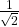and cos (A -B) =then ∠B =
A) 60°
B) 45°
C) 30°
D) 0°

D) 0°
Explanation: sin (A + B) == sin45°
A+B = 45°__________(1)
Cos(A-B) == cos45°
A-B = 45° ___________(2)
By Solving (1) and (2)
A + B = 45°
A-B = 45°
________
2A = 90°
________
A = 45°
∠B = 45°

Q). If sinθ + cosθ = √2 then θ =
A) 0°
B) 30°
C) 45°
D) 60°

C) 45°
Explanation: By options, take θ = 45°
then sin 45° + cos 45°
=+=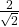= √2

Q).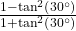=
A) 1/2
B) 1/√2
C) √3/2
D) 1

A) 1/2
Explanation: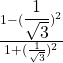=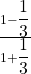=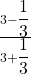= 2/4
= 1/2

Q). Cos4θ – Sin4θ =
A) Cos2θ – Sin2θ
B) 2 Cos2θ – 1
C) 1-2 Sin2θ
D) None

A) Cos2θ – Sin2θ
B) 2 Cos2θ – 1
C) 1-2 Sin2θ
Explanation: (cos2θ)2 -(sin2θ)2
=(cos2θ +sin2θ) (cos2θ -sin2θ)
=cos2θ -sin2θ or 1-2sin2θ or 2cos2θ-1

Q). The angle of elevation of the top of the cliff from a point 300 m from its foot is 60°. Then the height of the cliff is
A) 200√3
B) 200/√3
C) 300√3
D) 300/√3

C) 300√3
Explanation: Tan60° = AB/BC ⇒ √3 = AB/300
⇒ AB = 300√3

Q). The angle of elevations of the top of a tower from the top and foot of a pole of height 10 m are 30° and 60°. Then the height of the tower is
A) 5 m
B) 10 m
C) 15 m
D) 20 m

C) 15 m

Q). The set of all possible events is called………
A) event
B) impossible event
C) sample space
D) None

C
Explanation:

Q). From a deck of 52 cards, a card is drawn at random, then the probability of getting a red king is
A) 1/26
B) 2/13
C) 3/26
D) None

A) 1/26
Explanation: No. of cards = 52
Red card = 2 (1-heart, 1-diamond)
probability of getting red card
= 2/52
= 1/26

Q). If P(A)= 4/15 = then P A)
A) 19/15
B) 11/15
C) 13/15
D) None

B) 11/15
Explanation: PA) =1-P(A)
= 1- 4/15
= 15-4/15
= 11/15

Q). The probability of getting 53 Fridays in a Leap year is
A) 1/7
B) 2/7
C) 3/7
D) 4/7